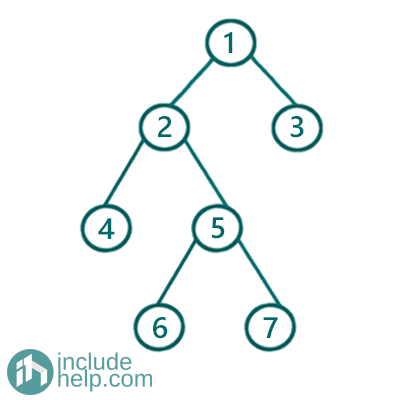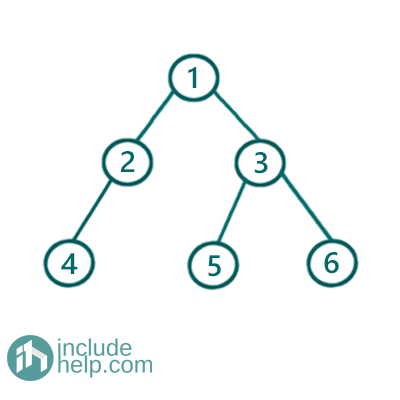# Check if a tree is full binary tree or not

In this article, we are going to see how to check two trees are structurally similar or not?
Submitted by Radib Kar, on August 07, 2020

In the tutorial on Full binary Tree, we saw that, a Full Binary Tree is a special kind of binary tree where each node has either 2 children or 0 children (leaf nodes).

For example the below binary tree is a full binary tree whereas the second one is not.

Example 1:Figure 1: Full Binary Tree

The above example is a Full Binary Tree as every node in this, either has two children or no children.

Example 2:Figure 2: Binary Tree which is Not a Full Binary Tree

The above binary tree is not a full binary tree as all its nodes don't have either 2 or 0 children. The node having value 2 violates the condition as it has single child only (4). Don't think NULL is a child!

Now to check whether A tree is Full binary Tree or not we can use both recursive & iterative approach which are listed below:

1) Recursive implementation

Comments are there to show how it's working.

```#include <bits/stdc++.h>
using namespace std;

class TreeNode {
public:
int val;
TreeNode* left;
TreeNode* right;

TreeNode(int v)
{
val = v;
left = NULL;
right = NULL;
}
};

//Full Binary Tree checking recursively
bool is_Full_Binary_Tree(TreeNode* root)
{
if (!root)
return true;

//if its a leaf node then fine, return true
if (!root->left && !root->right) {
return true;
}

/*
if it has only one children then return False
since in the previous step, we had checked for that "&&"" condition
it's guaranteed that both is Not NULL if control reaches here
so "||" will actually check whether anyone is NULL and other one not
if both is not not NULL it's doesn't enter the loop*/

if (!root->left || !root->right) {
return false;
}

/*
if control reaches here that means the
current node has two children( Not NULL)
so now check whether the subtrees are FULL or NOT
both of the subtrees need to be Full binary tree
themselves and we can check that recursively
*/
return is_Full_Binary_Tree(root->left) && is_Full_Binary_Tree(root->right);
}

int main()
{
//Example 1 Tree is built
TreeNode* root1 = new TreeNode(1);
root1->left = new TreeNode(2);
root1->right = new TreeNode(3);
root1->left->left = new TreeNode(4);
root1->left->right = new TreeNode(5);
root1->left->right->left = new TreeNode(6);
root1->left->right->right = new TreeNode(7);

if (is_Full_Binary_Tree(root1))
cout << "The tree is Example 1 is a Full Binary Tree\n";
else
cout << "The tree is Example 1 is not a Full Binary Tree\n";

//Example 2 Tree is built
TreeNode* root2 = new TreeNode(1);
root2->left = new TreeNode(2);
root2->right = new TreeNode(3);
root2->left->left = new TreeNode(4);
root2->right->left = new TreeNode(5);
root2->right->right = new TreeNode(6);

if (is_Full_Binary_Tree(root2))
cout << "The tree in Example 2 is a Full Binary Tree\n";
else
cout << "The tree in Example 2 is not a Full Binary Tree\n";

return 0;
}
```

Output:

```The tree is Example 1 is a Full Binary Tree
The tree in Example 2 is not a Full Binary Tree
```

2) Using iterative Approach

Comments are there to show how it's working.

```#include <bits/stdc++.h>
using namespace std;

class TreeNode {
public:
int val;
TreeNode* left;
TreeNode* right;

TreeNode(int v)
{
val = v;
left = NULL;
right = NULL;
}
};

//Full Binary Tree checking iteratively
bool is_Full_Binary_Tree(TreeNode* root)
{
if (!root)
return true;

//do breadth first traversal
queue<TreeNode*> q;
q.push(root);
while (!q.empty()) {
TreeNode* temp = q.front();
q.pop();
//if leaf node
if (!temp->left && !temp->right)
continue;
//if current node has only one child and another is NULL
else if (!temp->left || !temp->right)
return false;
else { //it has both the left & right child, so push both
q.push(temp->left);
q.push(temp->right);
}
}

return true;
}

int main()
{
//Example 1 Tree is built
TreeNode* root1 = new TreeNode(1);
root1->left = new TreeNode(2);
root1->right = new TreeNode(3);
root1->left->left = new TreeNode(4);
root1->left->right = new TreeNode(5);
root1->left->right->left = new TreeNode(6);
root1->left->right->right = new TreeNode(7);

if (is_Full_Binary_Tree(root1))
cout << "The tree is Example 1 is a Full Binary Tree\n";
else
cout << "The tree is Example 1 is not a Full Binary Tree\n";

//Example 2 Tree is built
TreeNode* root2 = new TreeNode(1);
root2->left = new TreeNode(2);
root2->right = new TreeNode(3);
root2->left->left = new TreeNode(4);
root2->right->left = new TreeNode(5);
root2->right->right = new TreeNode(6);

if (is_Full_Binary_Tree(root2))
cout << "The tree in Example 2 is a Full Binary Tree\n";
else
cout << "The tree in Example 2 is not a Full Binary Tree\n";

return 0;
}
```

Output:

```The tree is Example 1 is a Full Binary Tree
The tree in Example 2 is not a Full Binary Tree
```

What's New (MCQs)

Top Interview Coding Problems/Challenges!

Languages: » C » C++ » C++ STL » Java » Data Structure » C#.Net » Android » Kotlin » SQL
Web Technologies: » PHP » Python » JavaScript » CSS » Ajax » Node.js » Web programming/HTML
Solved programs: » C » C++ » DS » Java » C#
Aptitude que. & ans.: » C » C++ » Java » DBMS
Interview que. & ans.: » C » Embedded C » Java » SEO » HR
CS Subjects: » CS Basics » O.S. » Networks » DBMS » Embedded Systems » Cloud Computing
» Machine learning » CS Organizations » Linux » DOS
More: » Articles » Puzzles » News/Updates

© https://www.includehelp.com some rights reserved.